# 978-0134292380 Chapter 6 Part 1

Document Type
Test Prep
Book Title
Fundamentals of Hydraulic Engineering Systems 5th Edition
Authors
A. Osman H. Akan, Ned H. C. Hwang, Robert J. Houghtalen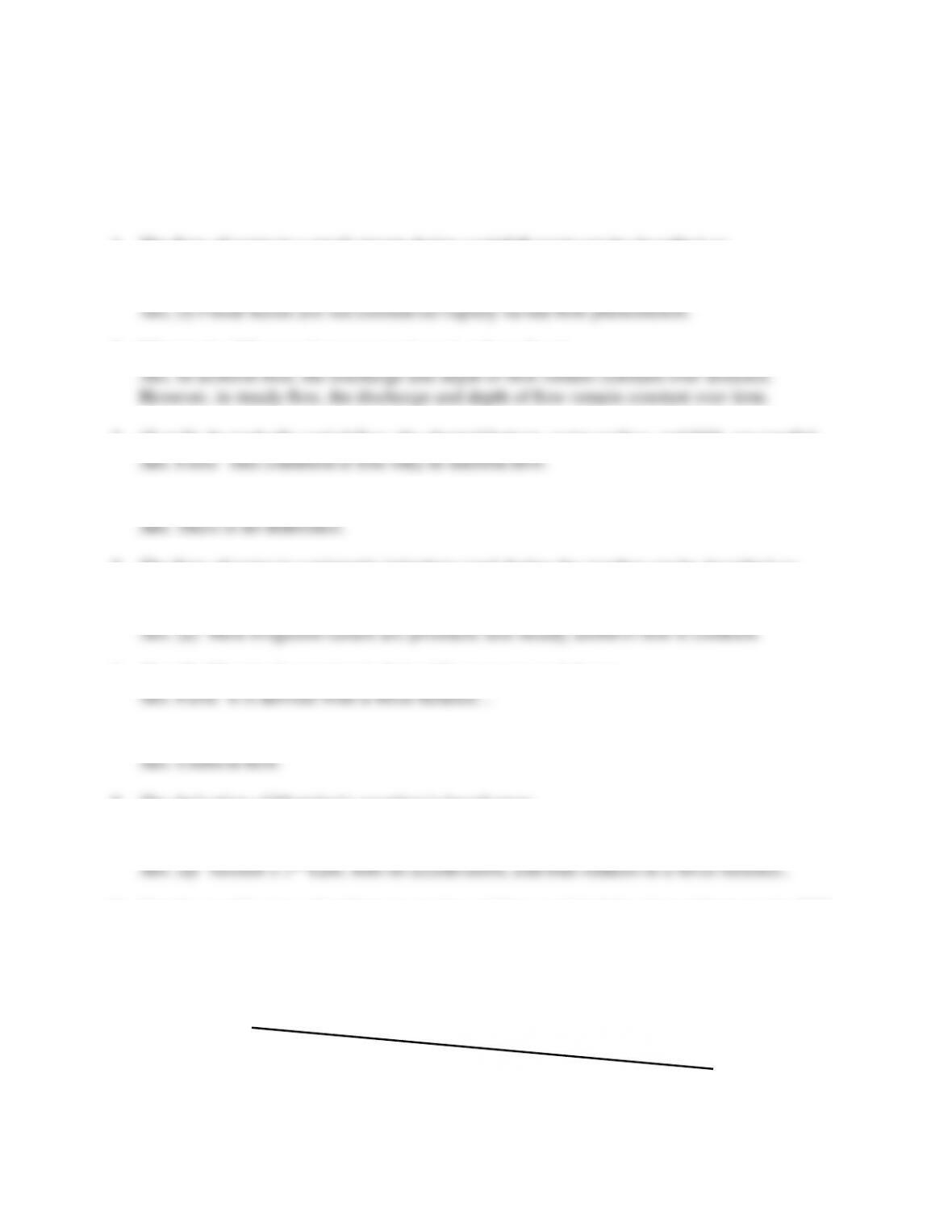54
TEST QUESTIONS & PROBLEMS - CHAPTER #6
1. The flow of water in a small stream during a rainfall event can be described as:
2. What is the difference between steady and uniform flow?
3. (T or F) In gradually varied flow, the channel bottom, water surface, and EGL are parallel.
4. What is the difference between normal depth and uniform depth?
5. The flow of water in a prismatic irrigation canal during dry weather can be described as:
6. (T or F) Manning’s equation is derived from an energy balance.
7. What type of flow regime is appropriate for the application of Manning’s equation?
8. The derivation of Manning’s equation is based upon:
a) Newton’s 2nd Law b) impulse-momentum principles c) Bernoulli principle
d) continuity principle e) Darcy-Wesibach
9. Sketch a profile view of uniform open channel flow and label the channel bottom, the HGL,
and the EGL. Also identify the three major components of energy on the figure.
Ans. See Figure 6.1 in the textbook.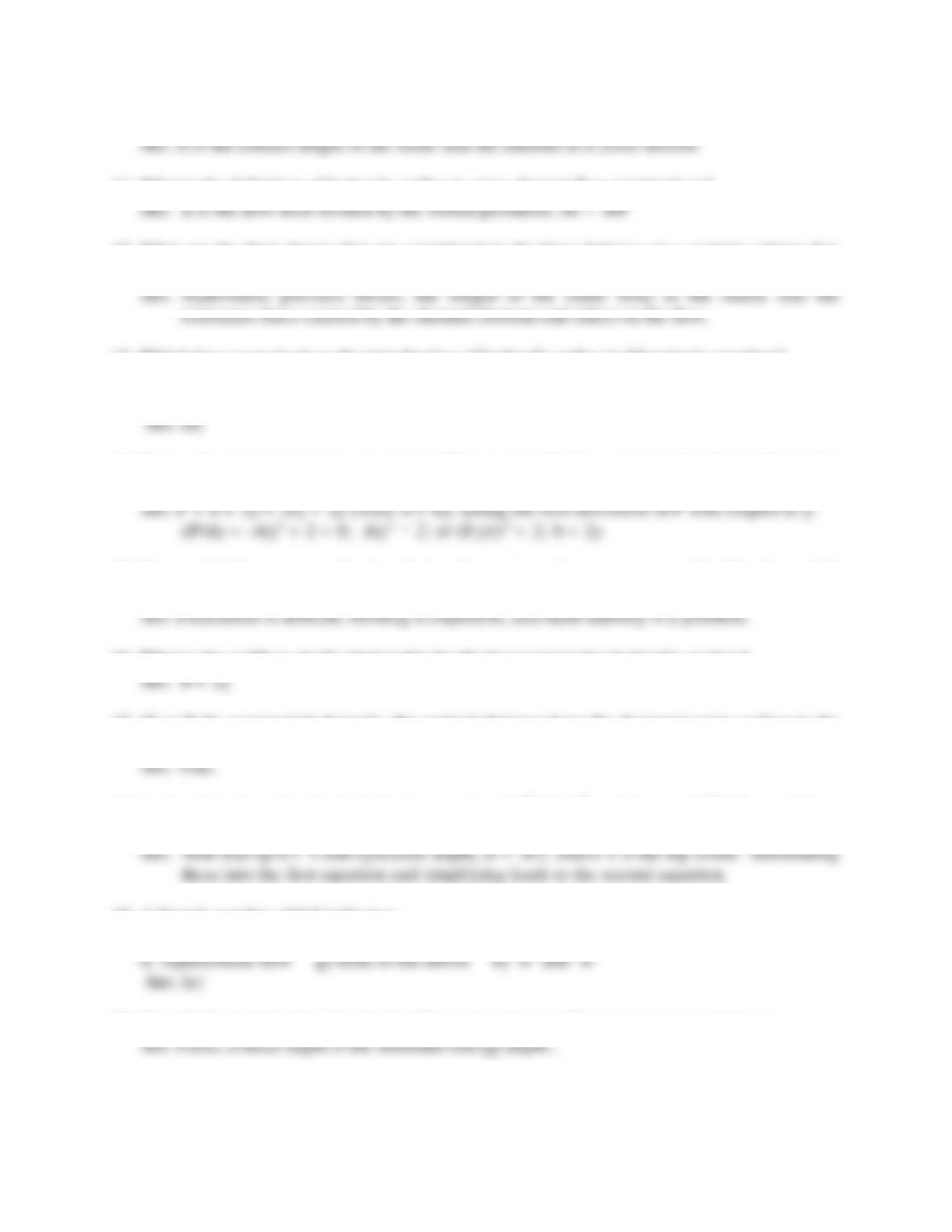55
10. What is the definition of wetted perimeter in open channel flow terminology?
11. What is the definition of hydraulic radius in open channel flow terminology?
12. What are the three forces that are considered in the force balance on a control volume that
leads to the development of Manning’s equation for uniform flow?
13. Which force term leads to the introduction of hydraulic radius in Manning’s equation?
a) hydrostatic pressure b) the weight of the water c) impulse-momentum
d) the resistance force exerted by the channel on the flow
14. Derive the relationship between depth of flow and width for a rectangular channel that is the
most hydraulically efficient (i.e., best hydraulic section). Show all steps.
15. If a semicircle represents the best hydraulic section, give two reasons why this shape isn’t
used for constructed water conveyance channels.
16. What is the width to depth relationship for the best rectangular hydraulic section?
17. (T or F) In constructed channels, the vertical distance from the designed water surface to the
top of the channel banks is known as the freeboard of the channel.
18. In the derivation of critical depth, the equation (Q2T)/(gA3) = 1 became V2/(gD) = 1. Show
the steps that led to the final equation and define T and D.
19. A Froude number of 0.8 indicates:
a) subcritical flow b) critical depth c) same as “e” d) normal depth e) there is no “e”
20. (T or F) Normal depth is the depth of flow when the specific energy is at a minimum.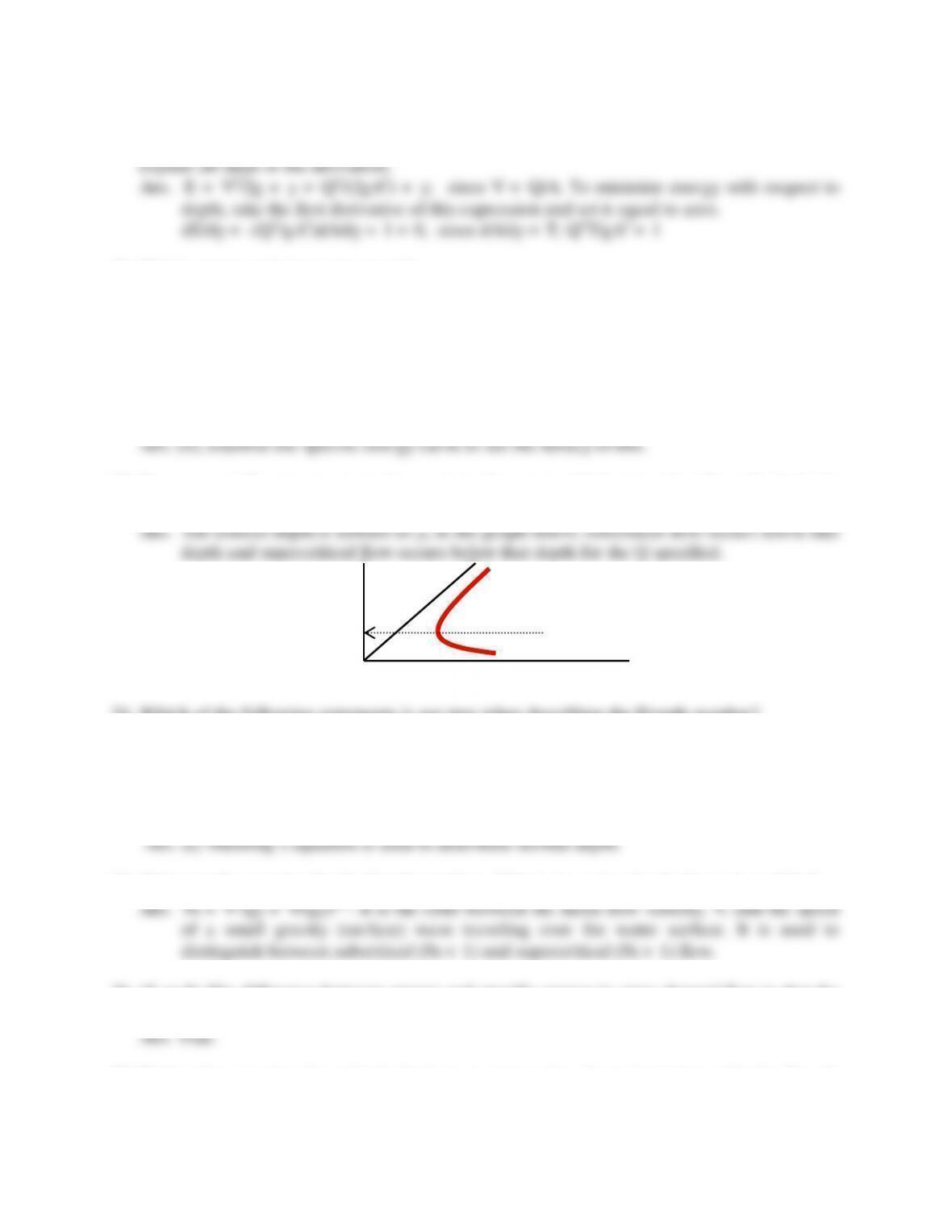56
21. Starting with the specific energy equation for open channel flow, E = V2/2g + y, show that
you will arrive at (Q2T)/(gA3) = 1 for critical depth (i.e., for minimum energy). Show and
22. Which statement below is incorrect?
(a) In a given channel the flow depth is higher than the critical depth for the same discharge
when the flow is subcritical
(b) In a given channel the specific energy for a given discharge is always higher for
supercritical flow than for subcritical flow.
(c) The critical depth in a channel for a given discharge does not depend on the longitudinal
bottom slope of the channel
(d) None of the above
23. Draw a specific energy curve for a given flow rate, label axes, identify critical depth,
subcritical flow, and supercritical flow.
24. Which of the following statements is not true when describing the Froude number?
a) The Froude number is the ratio of the mean flow velocity to the speed of a surface wave.
b) The Froude number is derived from the specific energy equation.
c) The Froude number is used to determine normal depth.
d) If Nf < 1, flow is subcritical, and if Nf > 1, flow supercritical.
e) The concept of top width is introduced in the derivation of the Froude number.
25. Write out the equation for the Froude number. What is it a ratio of and what is it used for?
26. (T or F) The difference between energy and specific energy in open channel flow is that the
specific energy uses the channel bottom as a datum (reference).
27. Derive the equation for critical depth in a rectangular channel starting with the Froude
number and setting it equal to unity: Nf = V2/gy = 1.
yc
subcritical
supercritical
Specific energy, E
Depth, y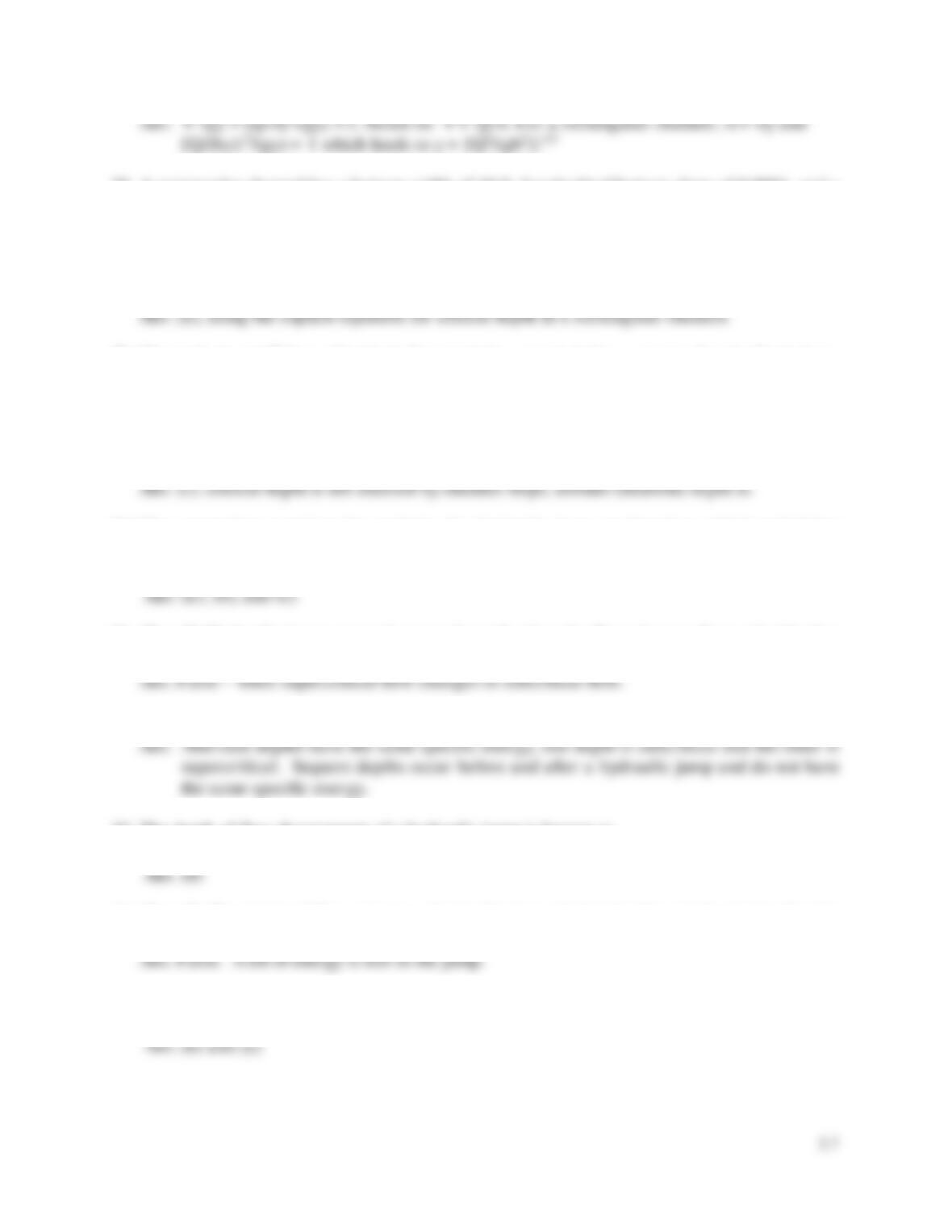57
28. A rectangular channel has a bottom width of 10 ft, longitudinal bottom slope of 0.0001, and a
Manning roughness factor of 0.016. If the critical depth in the channel is 1.91 ft
(a) The discharge in the channel is nearest 150 cfs
(b) The discharge in the channel is nearest 22 cfs
(c) The discharge in the channel is nearest 623 cfs
(d) Not enough information is given to determine the discharge.
29. Channels A and B have identical characteristics except A has a steeper longitudinal slope.
Determine which of the statements below is correct for the same discharge in both channels
(a) The critical depth in channel A is larger than the critical depth in channel B
(b) The critical depth in channel A is smaller than the critical depth in channel B
(c) The critical depth in channel A is same as the critical depth in channel B
(d) Critical flow does not occur in the steeper channel
30. The appropriate equations for analysis of a hydraulic jump are based on which underlying
concepts? a) energy principles b) momentum principles c) friction loss principles
d) uniform flow principles e) continuity principles
31. (T or F) Hydraulic jumps occur in open channels when the flow changes from subcritical to
supercritical.
32. What is the difference between alternate depth and sequent depth?
33. The depth of flow downstream of a hydraulic jump is known as
a) normal depth b) critical depth c) alternate depth d) sequent depth e) “a” and “d”
34. (T or F) The depth of flow prior to a hydraulic jump (initial depth) and the depth after the
jump (sequent depth) have the same specific energy.
35. The flow in a mild-sloped stream during a flood event can be described as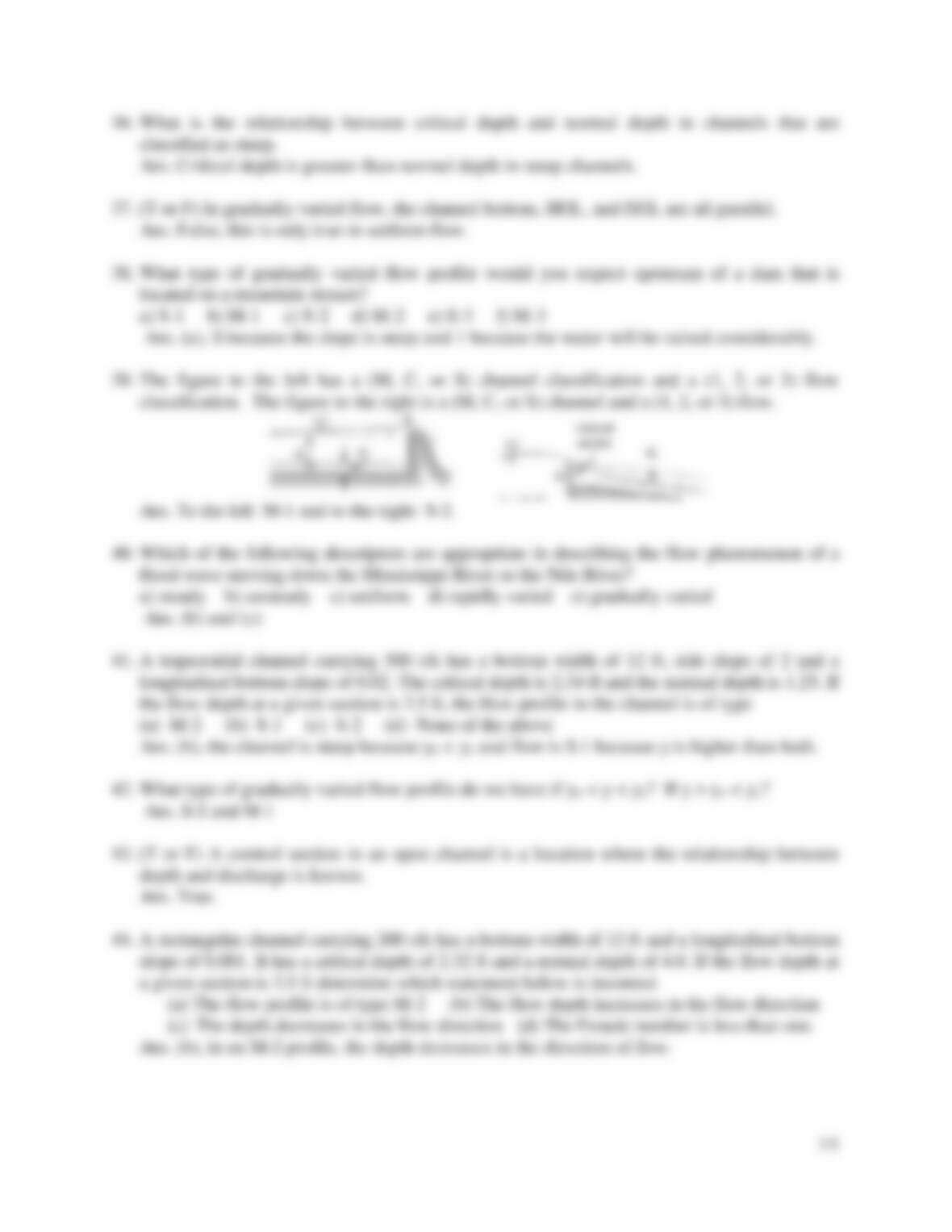36. What is the relationship between critical depth and normal depth in channels that are
classified as steep.
37. (T or F) In gradually varied flow, the channel bottom, HGL, and EGL are all parallel.
38. What type of gradually varied flow profile would you expect upstream of a dam that is
located on a mountain stream?
a) S-1 b) M-1 c) S-2 d) M-2 e) S-3 f) M-3
39. The figure to the left has a (M, C, or S) channel classification and a (1, 2, or 3) flow
classification. The figure to the right is a (M, C, or S) channel and a (1, 2, or 3) flow.
40. Which of the following descriptors are appropriate in describing the flow phenomenon of a
flood wave moving down the Mississippi River or the Nile River?
41. A trapezoidal channel carrying 300 cfs has a bottom width of 12 ft, side slope of 2 and a
longitudinal bottom slope of 0.02. The critical depth is 2.34 ft and the normal depth is 1.25. If
the flow depth at a given section is 3.5 ft, the flow profile in the channel is of type
(a) M-2 (b) S-1 (c) S-2 (d) None of the above
42. What type of gradually varied flow profile do we have if yn < y < yc? If y > yn < yc?
43. (T or F) A control section in an open channel is a location where the relationship between
depth and discharge is known.
44. A rectangular channel carrying 200 cfs has a bottom width of 12 ft and a longitudinal bottom
slope of 0.001. It has a critical depth of 2.32 ft and a normal depth of 4.0. If the flow depth at
a given section is 3.5 ft determine which statement below is incorrect
(a) The flow profile is of type M-2 (b) The flow depth increases in the flow direction
(c) The depth decreases in the flow direction (d) The Froude number is less than one.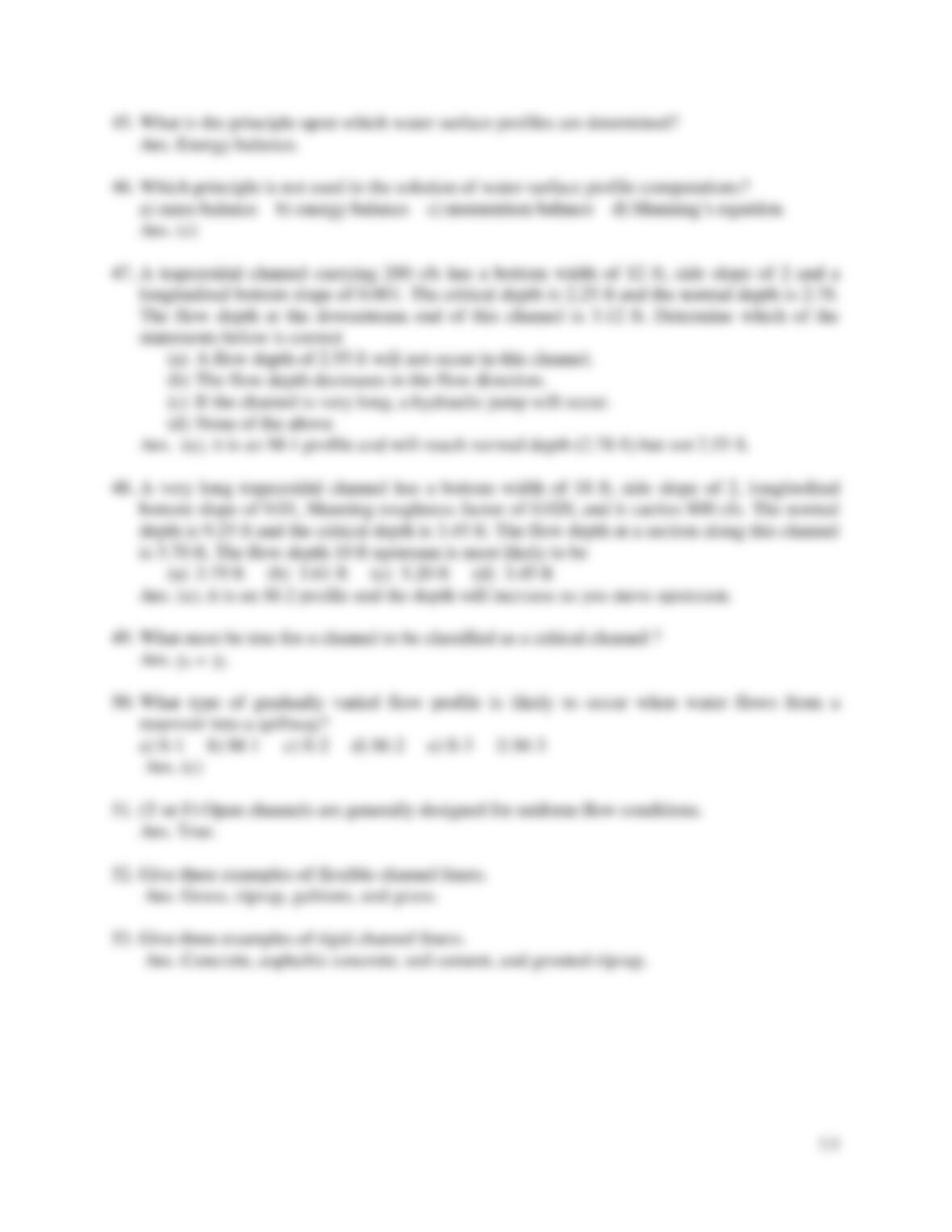45. What is the principle upon which water surface profiles are determined?
46. Which principle is not used in the solution of water surface profile computations?
a) mass balance b) energy balance c) momentum balance d) Manning’s equation
47. A trapezoidal channel carrying 280 cfs has a bottom width of 12 ft, side slope of 2 and a
longitudinal bottom slope of 0.001. The critical depth is 2.25 ft and the normal depth is 2.78.
The flow depth at the downstream end of this channel is 3.12 ft. Determine which of the
statements below is correct
(a) A flow depth of 2.55 ft will not occur in this channel.
(b) The flow depth decreases in the flow direction.
(c) If the channel is very long, a hydraulic jump will occur.
(d) None of the above
48. A very long trapezoidal channel has a bottom width of 18 ft, side slope of 2, longitudinal
bottom slope of 0.01, Manning roughness factor of 0.020, and it carries 800 cfs. The normal
depth is 9.25 ft and the critical depth is 3.45 ft. The flow depth at a section along this channel
is 3.70 ft. The flow depth 10 ft upstream is most likely to be
(a) 3.79 ft (b) 3.61 ft (c) 5.20 ft (d) 3.45 ft
49. What must be true for a channel to be classified as a critical channel ?
Ans. yn = yc
50. What type of gradually varied flow profile is likely to occur when water flows from a
reservoir into a spillway?
a) S-1 b) M-1 c) S-2 d) M-2 e) S-3 f) M-3
51. (T or F) Open channels are generally designed for uniform flow conditions.
52. Give three examples of flexible channel liners.
53. Give three examples of rigid channel liners.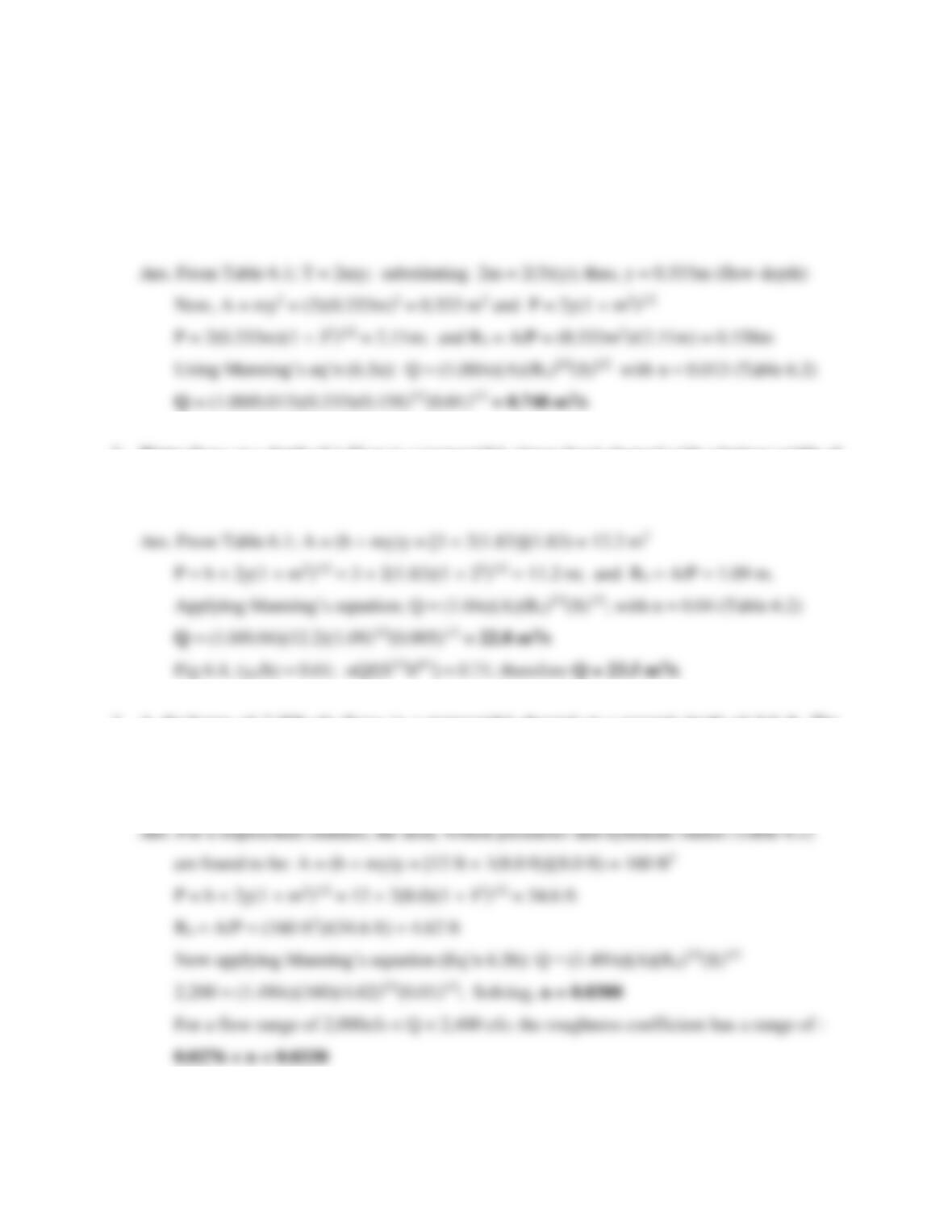60
Problems
1. A triangular roadside channel has side slopes of 3:1 (H:V) and a longitudinal slope of 0.01.
Determine the flow rate in the concrete channel if uniform flow is assumed when the top
width of the flowing water is 2 meters.
2. Water flows at a depth of 1.83 m in a trapezoidal, riprap lined channel with a bottom width of
3m, side slopes of 2:1 (H:V), and a bottom slope of 0.005. Determine the discharge in the
channel assuming uniform flow. Check your solution using Figure 6.4.
3. A discharge of 2,200 cfs flows in a trapezoidal channel at a normal depth of 8.0 ft. The
channel has a slope of 0.01, a 12 ft bottom width, and side slopes of 1:1 (H:V). Determine the
Manning coefficient of channel roughness. Also determine the sensitivity range of the
roughness coefficient estimate if the flow rate can only be estimated to within ±200 cfs?

## Trusted by Thousands ofStudents

Here are what students say about us.